## The Rank Plus Nullity Theorem

Let A be a matrix. Recall that the dimension of its column space (and row space) is called the rank of A. The dimension of its nullspace is called the nullity of A. The connection between these dimensions is illustrated in the following example.

Example 1: Find the nullspace of the matrix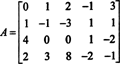The nullspace of A is the solution set of the homogeneous equation A x = 0. To solve this equation, the following elementary row operations are performed to reduce A to echelon form:Therefore, the solution set of A x = 0 is the same as the solution set of Ax = 0:With only three nonzero rows in the coefficient matrix, there are really only three constraints on the variables, leaving 5 − 3 = 2 of the variables free. Let x 4 and x 5 be the free variables. Then the third row of A′ implies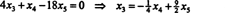The second row now yieldsfrom which the first row givesTherefore, the solutions of the equation A x = 0 are those vectors of the form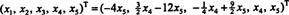To clear this expression of fractions, let t 1 = ¼ x 4 and t 2 = ½ x 5 then, those vectors x in R 5 that satisfy the homogeneous system A x = 0 have the form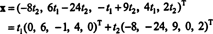Note in particular that the number of free variables—the number of parameters in the general solution—is the dimension of the nullspace (which is 2 in this case). Also, the rank of this matrix, which is the number of nonzero rows in its echelon form, is 3. The sum of the nullity and the rank, 2 + 3, is equal to the number of columns of the matrix.

The connection between the rank and nullity of a matrix, illustrated in the preceding example, actually holds for any matrix: The Rank Plus Nullity Theorem. Let A be an m by n matrix, with rank r and nullity ℓ. Then r + ℓ = n; that is,

rank A + nullity A = the number of columns of A

Proof. Consider the matrix equation A x = 0 and assume that A has been reduced to echelon form, A′. First, note that the elementary row operations which reduce A to A′ do not change the row space or, consequently, the rank of A. Second, it is clear that the number of components in x is n, the number of columns of A and of A′. Since A′ has only r nonzero rows (because its rank is r), n − r of the variables x 1, x 2, …, x n in x are free. But the number of free variables—that is, the number of parameters in the general solution of A x = 0—is the nullity of A. Thus, nullity A = n − r, and the statement of the theorem, r + ℓ = r + ( nr) = n, follows immediately.

Example 2: If A is a 5 x 6 matrix with rank 2, what is the dimension of the nullspace of A?

Since the nullity is the difference between the number of columns of A and the rank of A, the nullity of this matrix is 6 − 2 = 4. Its nullspace is a 4‐dimensional subspace of R 6.

Example 3: Find a basis for the nullspace of the matrix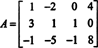Recall that for a given m by n matrix A, the set of all solutions of the homogeneous system A x = 0 forms a subspace of R n called the nullspace of A. To solve A x = 0, the matrix A is row reduced:Clearly, the rank of A is 2. Since A has 4 columns, the rank plus nullity theorem implies that the nullity of A is 4 − 2 = 2. Let x 3 and x 4 be the free variables. The second row of the reduced matrix givesand the first row then yields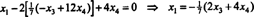Therefore, the vectors x in the nullspace of A are precisely those of the formwhich can be expressed as follows:If t 1 = 1/7 x 3 and t 2 = 1/7 x 4, then x = t 1(−2, −1, 7, 0) T + t 2(−4, 12, 0, 7) T, soSince the two vectors in this collection are linearly independent (because neither is a multiple of the other), they form a basis for N(A)